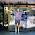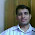## Monday, October 17, 2011

I read Jason Rosenhouse's book about The Monty Hall Problem recently, and I use the problem as an example in my statistics class.  Last semester I wrote a variation of the problem that turns out to be challenging, and a motivating problem for Bayesian estimation.  Here's what I call the "Blinky Monty Problem."
Suppose you are on Let's Make a Deal and you are playing the Monty Hall Game, with one twist.  Before you went on the show you analyzed tapes of previous shows and discovered that Monty has a tell: when the contestant picks the correct door, Monty is more likely to blink.
Of the 18 shows you watched, the contestant chose the correct door 5 times, and Monty blinked three of those times.  Of the other 13 times, Monty blinked three times.
Assume that you choose Door A.  Monty opens door B and blinks.  What should you do, and what is your chance of winning?

To get started, let's review the standard version of the Monty Hall problem (from Wikipedia):
"Suppose you're on a game show, and you're given the choice of three doors: Behind one door is a car; behind the others, goats. You pick a door, say A [but the door is not opened], and the host, who knows what's behind the doors, opens another door, say B, which has a goat. He then says to you, "Do you want to pick door C?" Is it to your advantage to switch your choice?"
We can solve this problem using Bayes's Theorem.  There are two relevant hypotheses:

A: the car is behind door A
C: the car is behind door C

Before Monty opens door B, the prior probability for both hypotheses is 1/3.  Now the evidence is

E: Monty opened door B.

To compute the posterior probabilities, we need the likelihoods P(E|A) and P(E|C).

P(E|A) is the probability of the evidence if the car is behind door A.  In this case Monty has a choice; he could have opened door B or C.  In the canonical version of the problem, he chooses at random, so

P(E|A) = 1/2

P(E|C) is the probability of the evidence if the car is behind door C.  In this case Monty has no choice, so

P(E|C) = 1

Applying Bayes's Theorem yields:

P(A|E) = 1/3
P(C|E) = 2/3

So if you switch to Door C, you increase the chances of winning from 1/3 to 2/3.  This is the standard answer to the standard version of the problem.  If you are not familiar with the Monty Hall problem and this answer comes as a shock to you, please don't take it out on me.  Go read about it, starting here, and come back when you have calmed down.

Are you ready to go on?  Ok.  We can do a similar analysis for the Blinky Monty problem, but now we have more evidence to consider:

E: Monty opens Door B and blinks.

According to the scouting report, the probability that Monty blinks if the car is behind A is 3/5, so

P(E|A) = (1/2)(3/5) = 3/10

If the car is behind C, Monty blinks 3/13 of the time, so

P(E|C) = (1)(3/13) = 3/13

Plugging in Bayes' Theorem, we get P(A|E) = 0.51, so in this scenario you are slightly better off sticking with Door A.

But is that all there is to it?  No!  And here's where this blog earns the name "Probably Overthinking It." Remember that the probabilities for Monty's blinking are estimates based on a sample, so we have some uncertainty about them.

To take that uncertainty into account, we have to

1) Use the scouting report to estimate the distribution of P(blink|A) and P(blink|C).

2) For each pair of values from those distributions, compute P(A|E).

3) Apply the law of total probability to get the overall P(A|E).

That might sound complicated, but it is straightforward to estimate computationally.

First, let me take a minute to make fun of frequentists.  According to conventional hypothesis testing, the data from the scouting report is not statistically significant.  If the null hypothesis is that Monty has the same chance of blinking regardless of the location of the car, the p-value of the sample we saw is 0.27 (I used Fisher's exact test, computed using this online calculator).  We can't reject the null hypothesis, so if we play by the rules of conventional hypothesis testing, I guess that means we can't take advantage of Monty's tell.  If you are a committed frequentist, you should stop reading now.

Good.  Now that the riff-raff are gone, let's proceed.  Here's how we make the posterior distribution for P(blink|doorA):

doorA = MakeUniformSuite(0.0, 1.0, 101, name='Door A')
evidence = 3, 2
Update(doorA, evidence)

MakeUniformSuite() makes a Pmf of 101 values equally spaced between 0 and 1, with equal probability. Update() does the usual Bayesian update:

def Update(suite, evidence):
"""Updates a suite of hypotheses based on new evidence.

Modifies the suite directly; if you want to keep the original, make
a copy.

Args:
suite: Pmf object
evidence: whatever kind of object Likelihood expects
"""
for hypo in suite.Values():
likelihood = Likelihood(evidence, hypo)
suite.Mult(hypo, likelihood)
suite.Normalize()

def Likelihood(evidence, hypo):
"""Computes the likelihood of the evidence assuming the hypothesis is true.

Args:
evidence: a tuple of (number of heads, number of tails)

Returns:
probability of tossing the given number of heads and tails with a
coin that has p probability of heads
"""
p = hypo
return pow(p, heads) * pow(1-p, tails)

If you are not familiar with that, you can read Chapter 8 of Think Stats, or see this blog post.

To find P(blink|C), it's pretty much the same:

doorC = MakeUniformSuite(0.0, 1.0, 101, name='Door C')
evidence = 3, 10
Update(doorC, evidence)

And to finish it off, we apply the law of total probability:

print TotalProbability(doorA, doorC, ProbWinning)

TotalProbability() enumerates the values from doorA and doorC, and calls ProbWinning() for each pair:

def TotalProbability(pmf1, pmf2, func):
"""Enumerates pairs from the Pmfs, calls the func, and returns
the total probability.

func: takes a value from each Pmf and returns a probability.
"""
total = 0.0
for x, px in pmf1.Items():
for y, py in pmf2.Items():
if px and py:
total += px * py * func(x, y)

def ProbWinning(pbA, pbC):
"""Computes the probability that the car is behind door A:

pbA: probability that Monty blinks if the car is behind A
pbC: probability that Monty blinks if the car is behind C
"""
pea = 0.5 * pbA
pec = 1.0 * pbC

pae = pea / (pea + pec)
return pae

The most likely values are 0.6 and 0.23, but since we don't have much data, the uncertainty is still large.
Taking it all together, the probability that the car is behind Door A, P(A|E), is 0.52.  So you are slightly better off sticking with Door A.

To summarize:

1) In the canonical version of the game, P(A|E) = 1/3, so you are better off switching.

2) In the Blinky Monty version, if we take P(blink|A) and P(blink|C) as givens, we estimate P(A|E) = 0.51, so you are slightly better off sticking with Door A.

3) If you are a frequentist, you conclude that Monty's tell is not statistically significant, so you ignore it, switch, and lose most of the time.

4) If you are a Bayesian, you can take advantage of the tell and maximize your chance of winning.  If Monty blinks and you stick, you win 52% of the time.  If he doesn't blink and you switch, you win 87% of the time.  Since he blinks 40% of the time, overall you can expect to win 73% of the time.  So the tell provides a substantial advantage.

Exercise for the reader: download my code and modify it to analyze the case where Monty doesn't blink.  Hint: you only have to change two lines.

EDIT October 19, 2011: I got a comment on reddit that raises a legitimate concern about data collection. In the scenario I presented, if you review tapes of the show and look for several potential tells (blinking, facial tics, verbal habits, etc.) and you choose one that seems to be correlated with the outcome, you could easily get fooled (see this post on repeated tests).  This is a fair warning for both the frequentist and Bayesian analyses.

So to be more careful, maybe I should say that you were tipped off to the possibility that Monty's tell is a blink before you reviewed the tape, and that's the only data you collected.

EDIT January 26, 2012: In the comments below, Jacob pointed out an error in my calculation.  As a result, I went back and changed the scenario to match my calculations, which forced me to make a few other revisions.  I think it is all settled now, but let me know if you disagree.

1.I believe that you made a mistake calculating P(A|E).

You write that you set: "evidence = 3, 10" when it should be "3, 7". Now, maybe that was just a typo, but in your graph, the "Door C" curve appears to peak, not around 0.3, but somewhere closer to 0.25 or maybe exactly 3/13 which would be the case setting your evidence to be "3, 10"

1.You are correct. I have revised the article accordingly (see the note at the end). Thanks very much!

2.We see P(E|A) = 1, instead it should be P(E|C) = 1

1.Fixed. Thank you!